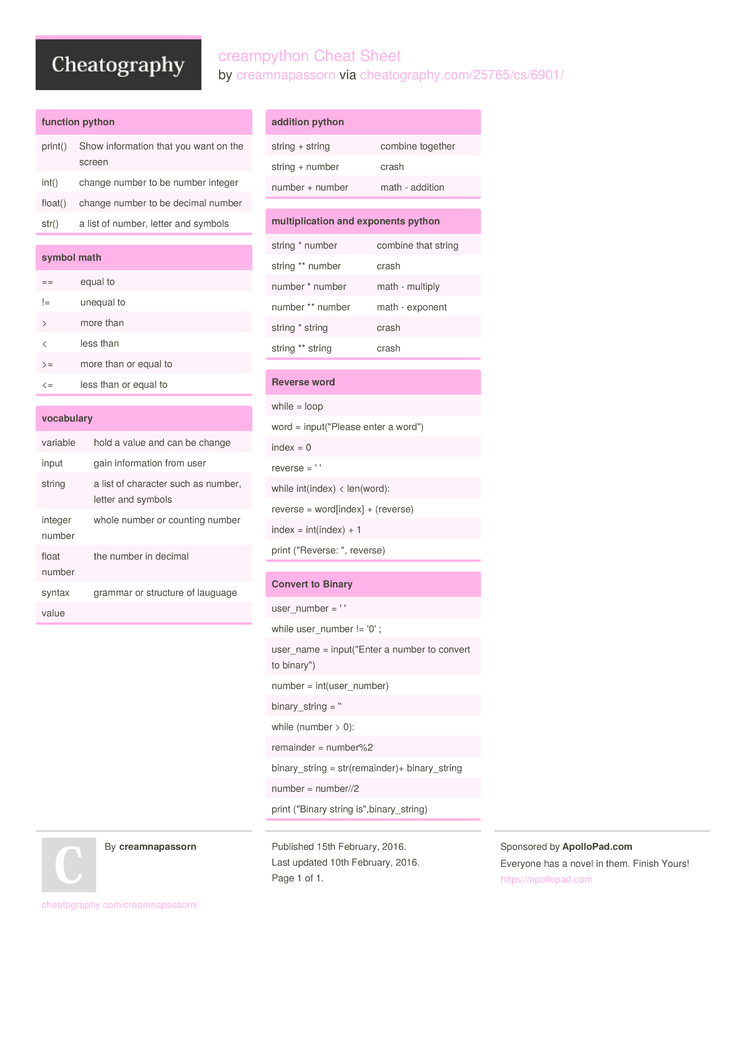# creampython Cheat Sheet by creamnapassorn

### function python

 print() Show inform­­ation that you want on the screen int() change number to be number integer float() change number to be decimal number str() a list of number, letter and symbols

### symbol math

 == equal to != unequal to > more than < less than >= more than or equal to <= less than or equal to

### vocabulary

 variable hold a value and can be change input gain inform­­ation from user string a list of character such as number, letter and symbols integer number whole number or counting number float number the number in decimal syntax grammar or structure of lauguage value

 string + string combine together string + number crash number + number math - addition

### multip­lic­ation and exponents python

 string * number combine that string string ** number crash number * number math - multiply number ** number math - exponent string * string crash string ** string crash

### Reverse word

 while = loop word = input(­"­Please enter a word") index = 0 reverse = ' ' while int(index) < len(word): reverse = word[i­ndex] + (reverse) index = int(index) + 1 print ("Re­verse: ", reverse)

### Convert to Binary

 user_n­umber = ' ' while user_n­umber != '0' ; user_name = input(­"­Enter a number to convert to binary­") number = int(us­er_­number) binary­_string = '' while (number > 0): remainder = number%2 binary­_string = str(re­mai­nder)+ binary­_string number = number//2 print ("Binary string is",­bin­ary­_st­ring)1 Page
//media.cheatography.com/storage/thumb/creamnapassorn_creampython.750.jpg

PDF (recommended)